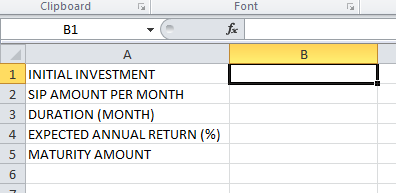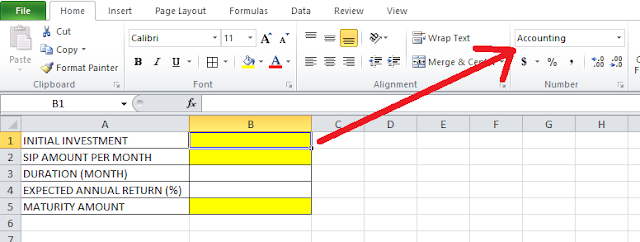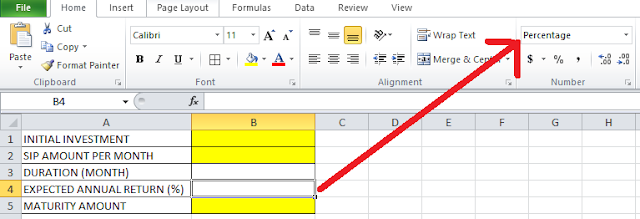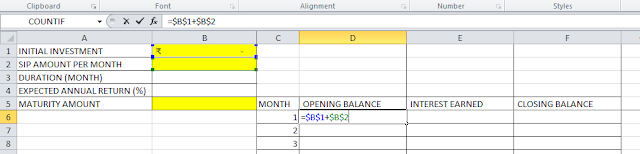# Lesson #13: Make a perfect SIP Planner in Excel

Hello friends! in this post I will give you an example of making a SIP planner in Excel. As you know there are so many apps in market to calculate SIP (Systematic Investment Plan) but it would be nice if you can build a spreadsheet just like that using same logic and calculation.At first I am going to make the field in which I have to enter data. That includes “INTIAL INVESTMENT”, “SIP AMOUNT PER MONTH”, “DURATION IN MONTH”, “EXPECTED ANNUAL RETURN” and “MATURITY AMOUNT” will be calculated and will be shown in the cell beside from above data.Those cells showing amount are put in Accounting format.Expected annual return shown in Percentage format.Now you have to put Duration in month which have done with Data Validation. I have allowed whole number only from 0 to 600 so a maximum  duration of 50 years can be calculated.Now I have made the chart on which the SIP will be calculated. It will be like this shown in the above picture. In first row for Opening balance I have put this formula =\$B\$1+\$B\$2 [Initial investment+SIP amount per month]. “\$” is given before both row and column so the cell reference always stay locked.

At the first row of interest earned I put the formula =\$D6*(\$B\$4/12) [Opening balance*(Annual return/12)]. Here I have put “\$” Before the column no of opening balance only so when I will drag the formula downward, reference of opening balance will also move downward in the same column. For the expected annual return I will take reference from the same cell so I have to put “\$” before both row and column.

Closing balance will be Opening balance+Interest earned which is =\$E6+\$D6.

Now in the second row opening balance I will put the formula of previous closing balance+SIP amount per month  by using formula =\$F6+\$B\$2. Then these formulas will be dragged down up to 600 no month.

In the Maturity amount cell I will put this formula =VLOOKUP(\$B\$3, \$C\$6:\$F\$605, 4,FALSE) which will look up the value of closing balance in SIP chart for the last month.

Look in the above picture this is how this sheet is perfectly working as a SIP calculator.

You may download the file SIP Planner in Excel from the link given below.

excelabcd-sip-planner

In future I will post more good examples of worksheets which can be made very easily with excel. I hope you liked my post and please don’t forget to share.Puspendu is the founder author of Excelabcd. He is a creative person, blogger and Excel-maniac guy.

Tagged with: ,